# Direction Cosines and Direction Ratios Solutions exercise 6(b)

Intermediate Mathematics 1B Solutions for textbook Exercise 6(b) Direction Cosines and Direction Ratios are given.

Study the textbook lesson Direction Cosines and Direction Ratios very well.

Observe the example problems and solutions given in the textbook. Try them.

Observe the given solutions and try them in your own method.

You can see the solutions for Inter Maths 1B

Maths 1B solutions for

Locus

Exercise 1(a)

Transformation of Axes

Exercise 2(a)

Three Dimensional Coordinates

Exercise 5(a)

Exercise 5(b)

Direction Cosines and Direction Ratios

Exercise 6(a)

The Plane

Exercise 7(a)

Limits and Continuity

Exercise 8(a)

Exercise 8(b)

Exercise 8(d)

Exercise 8(e)

M

# Intermediate Maths solutions for 1B Direction Cosines and Direction Ratios

Direction Cosines and Direction Ratios Solutions

Chapter 6 – Exercise 6(b)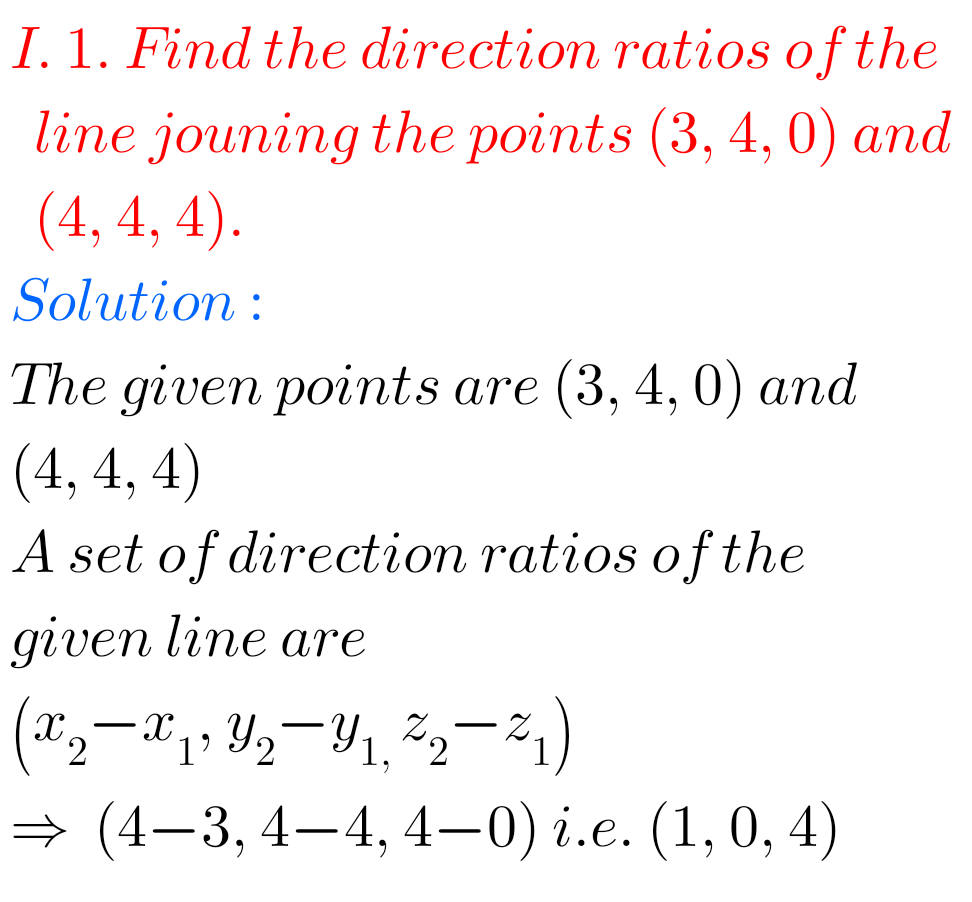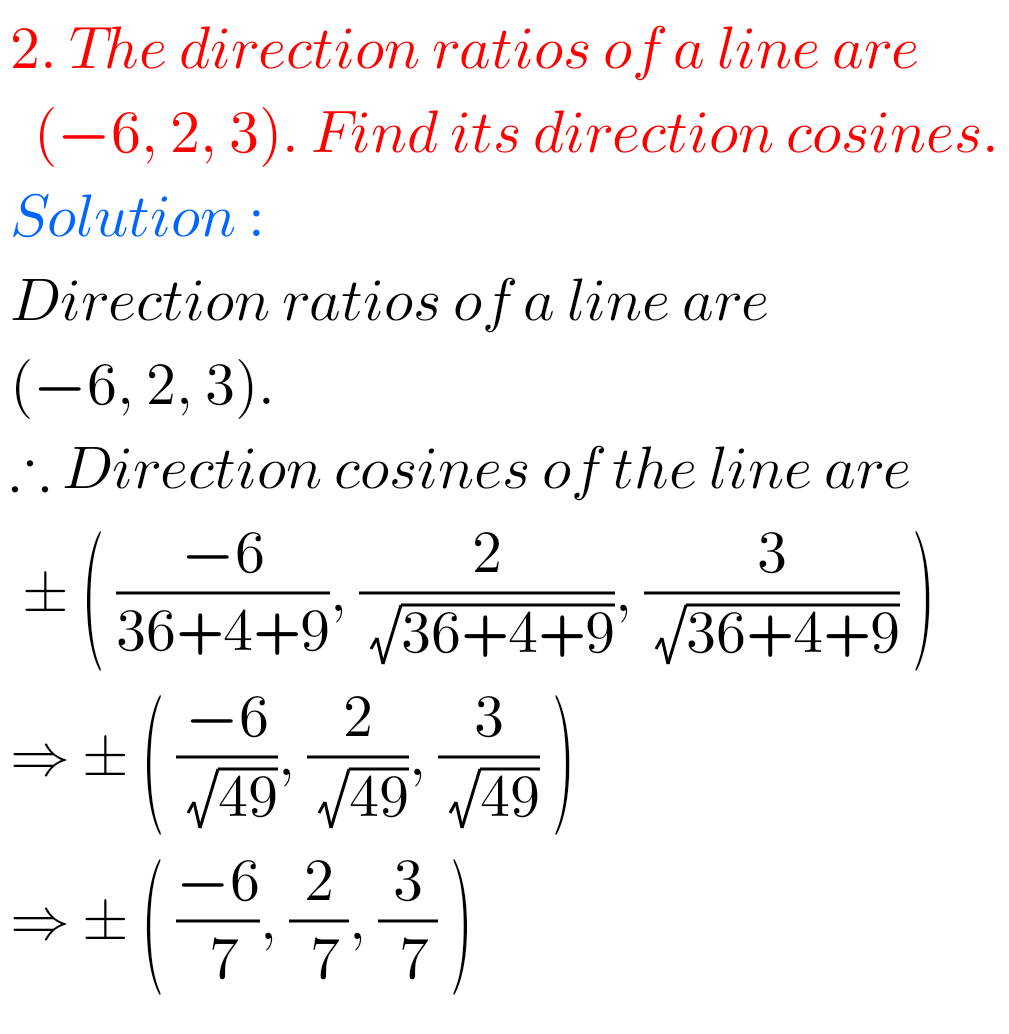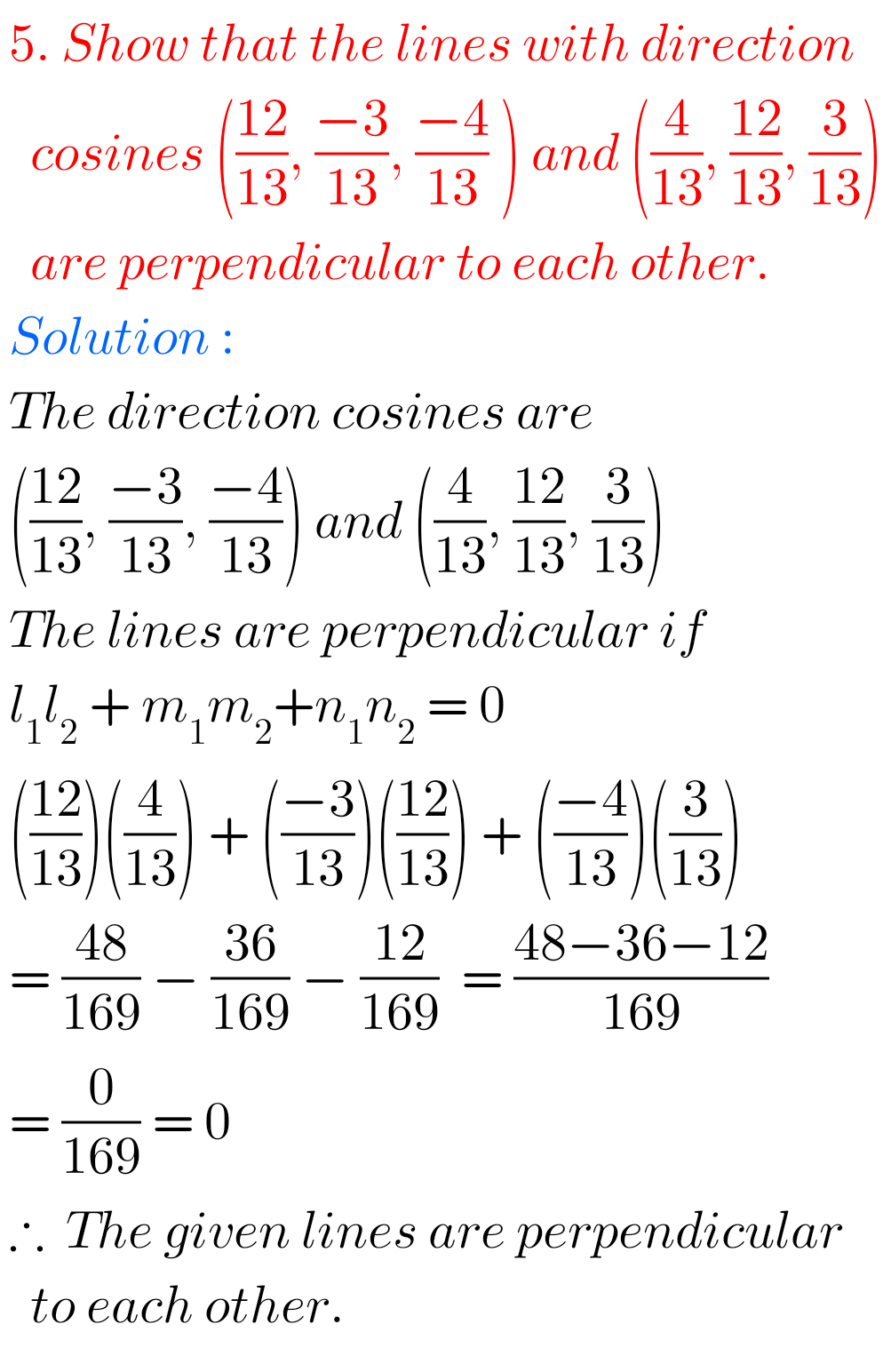## Maths Solutions for DC and DR Exercise 6(b) Inter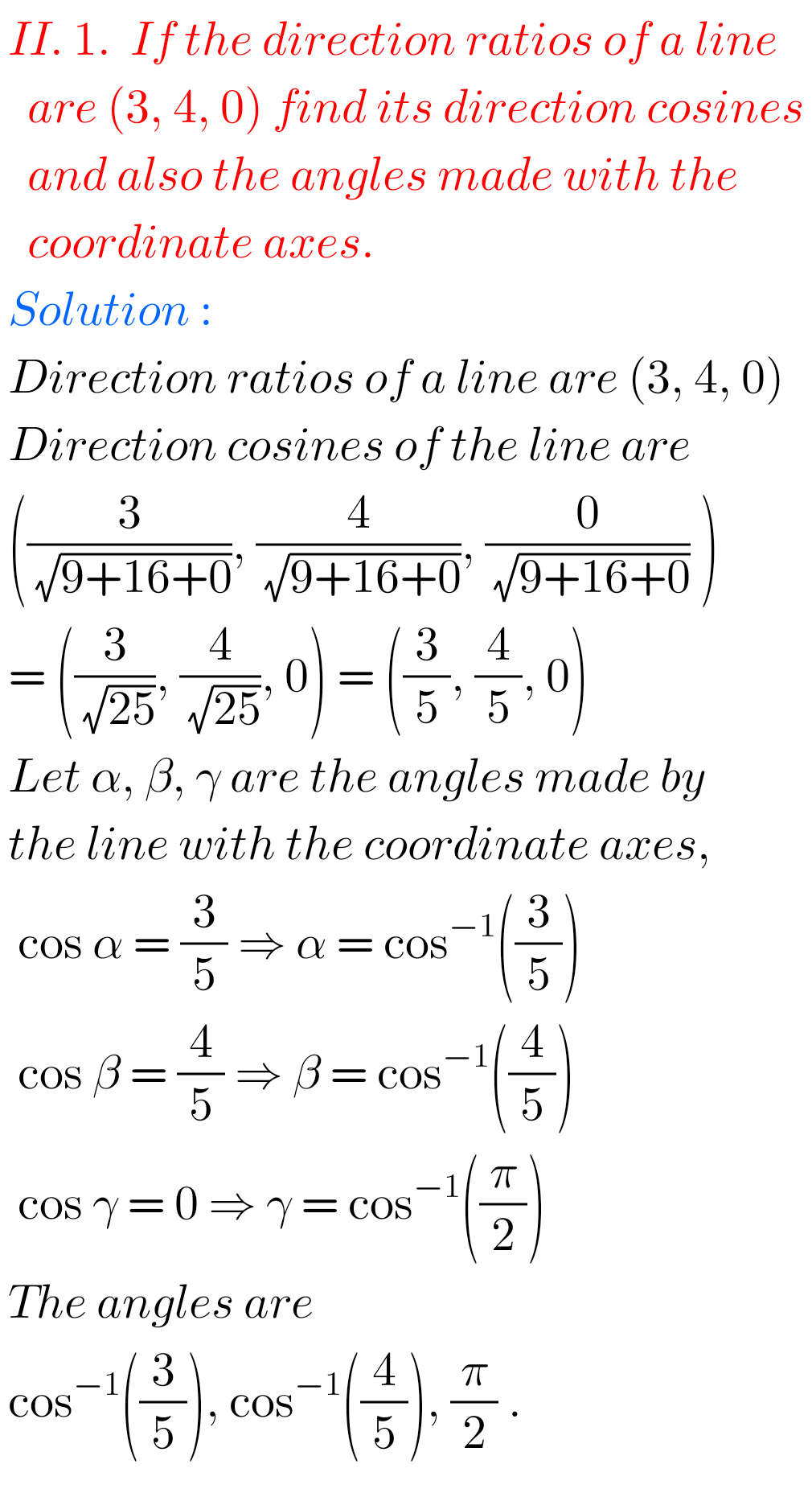## Solutions for Direction Cosines and Direction Ratios Exercise 6(b)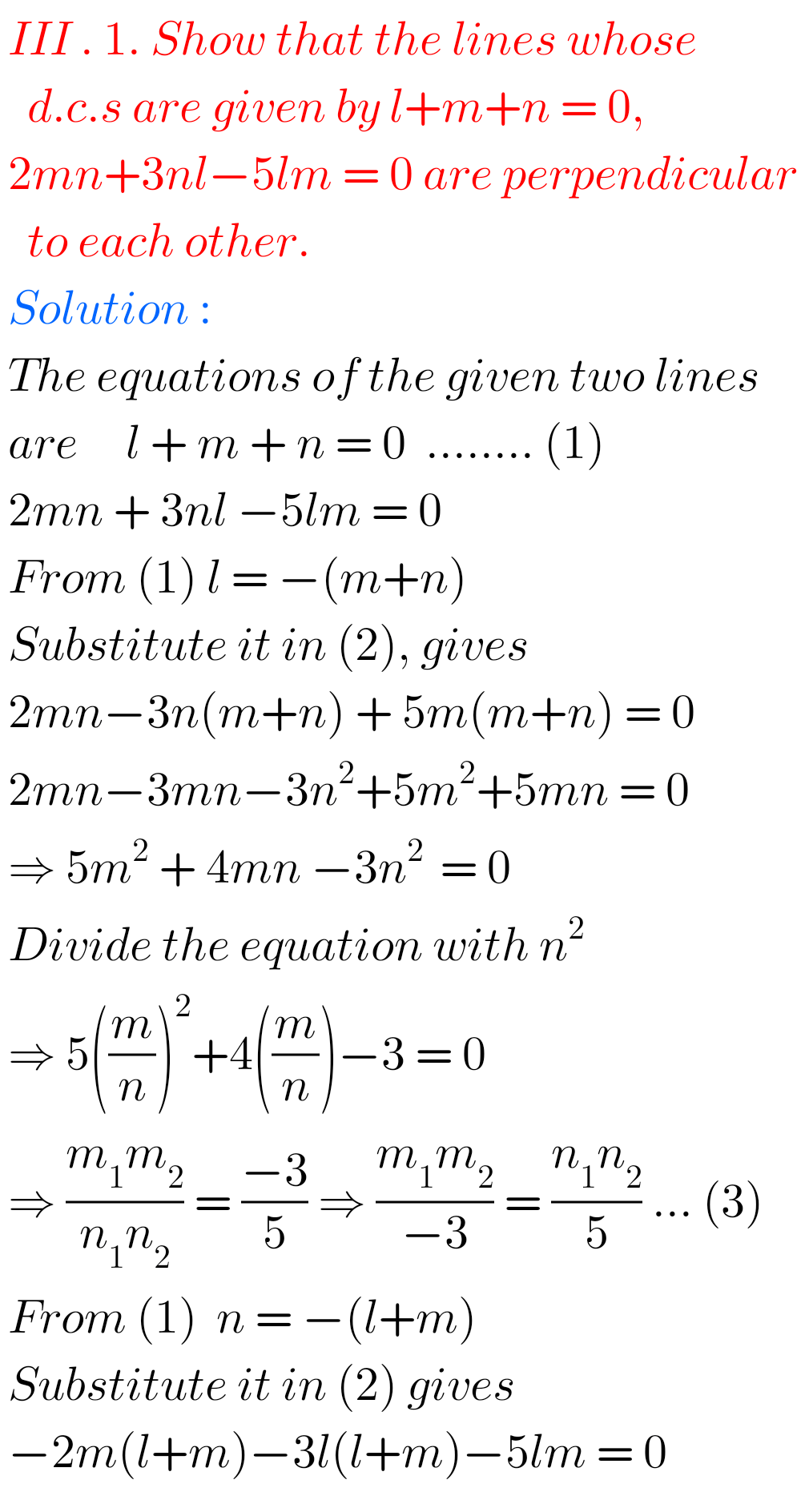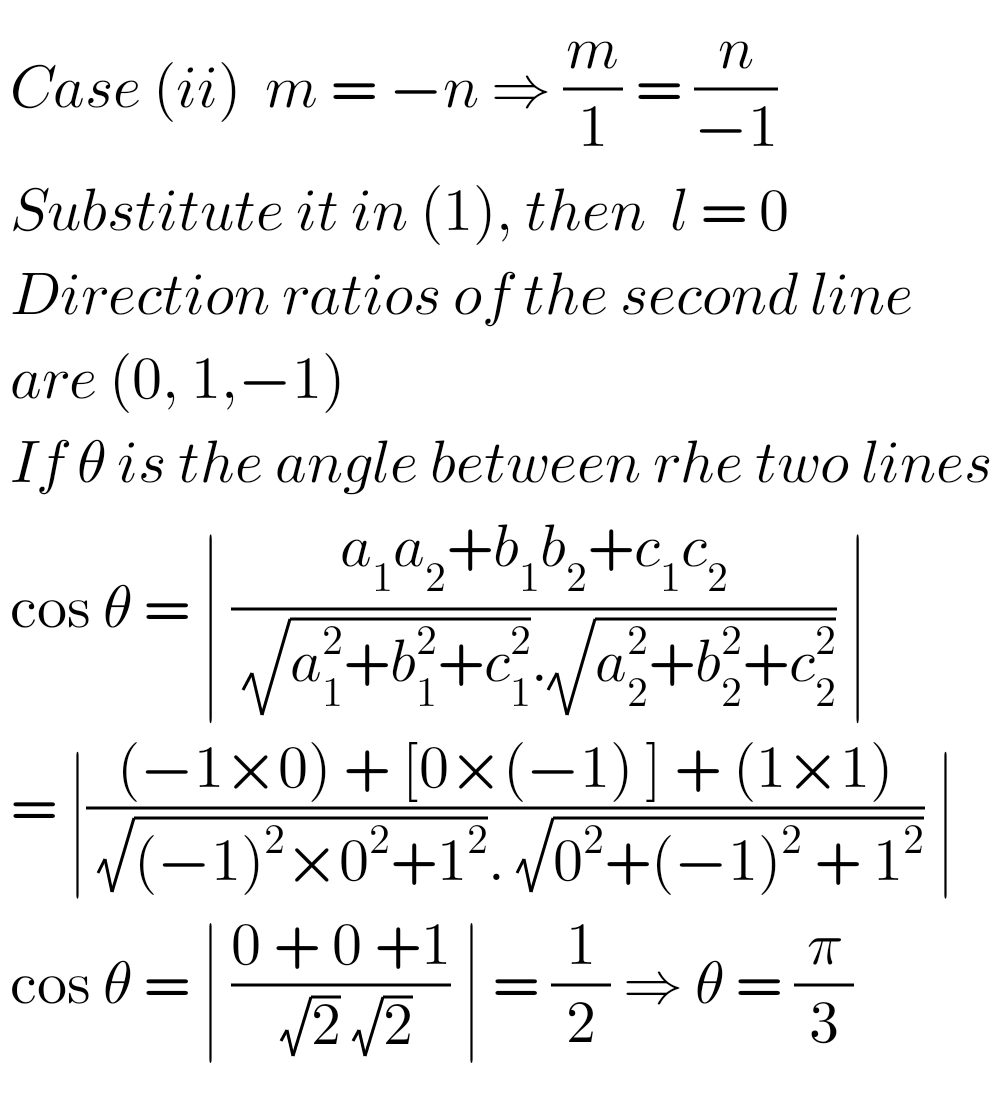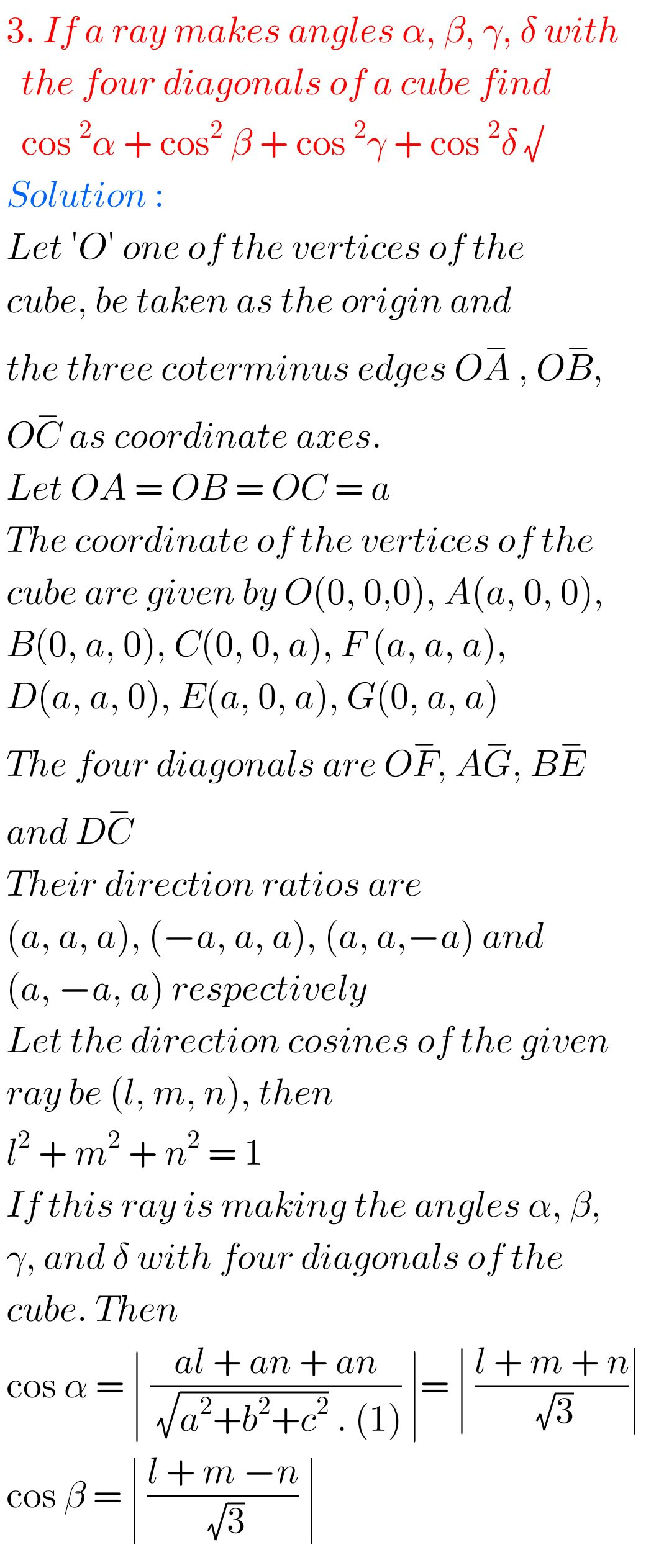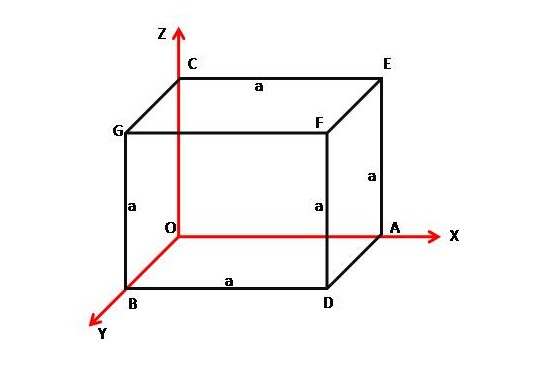M

Note: Observe the solutions and try them in your own method.

SSC Maths Class 10 Solutions

Maths IIA solutions Inter

Maths IIB solutions Inter

You can see the solutions for text book Maths 1A

Functions

Exercise 1(a)

Exercise 1(b)

Exercise 1(c)

Mathematical Induction

Exercise 2(a)

Matrices

Exercise 3(a)

Exercise 3(b)

Exercise 3(c)

Exercise 3(d)

Exercise 3(e)

Exercise 3(f)

Exercise 3(g)

Exercise 3(h)

Exercise 3(i)

Exercise 4(a)

Exercise 4(b)

Product of Vectors

Exercise 5(a)

Exercise 5(b)

Exercise 5(c)

Trigonometric Ratios up to Transformations

Exercise 6(a)

Exercise 6(b)

Exercise 6(c)

Exercise 6(d)

Exercise 6(e)

Exercise 6(f)

Trigonometric Equations

Exercise 7(a)

Inverse Trigonometric Equations

Exercise 8(a)

Hyperbolic Functions

Exercise 9(a)

Properties of Triangles

Exercise 10(a)

Exercise 10(b)